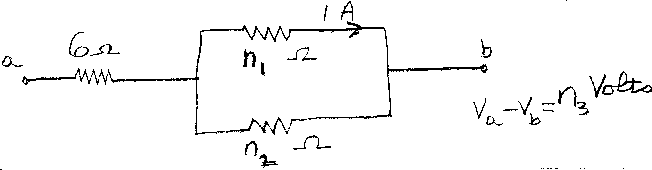Problem B2: Consider the three resistors in the figure above. One has a resistance of 6 Ohms, and the other two have resistances of n1 and n2 Ohms. If 1 Amp flows through the n1 Ohm resistor, what is the voltage difference between point a and point b. That is, what is n3 in the expression V(a) - V(b) = n3 Volts?n1 = n2 = Input n3:
If you are currently in my class, you can record your grade by entering your name and student ID number (without the leading zeros) below and clicking on "record grade".
 First Name = Last Name = ID = Problem: